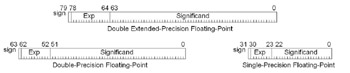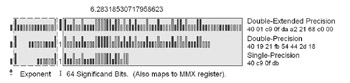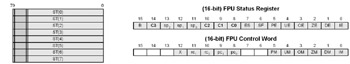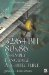# The Floating-Point NumberBefore digging very deeply let us first examine the floating-point number and its sub- components .Figure 8-1: Floating-point formats

The FPU supports three sizes of floating-point numbers , as shown below.

Data Size

C reference

Assembler

Bytes

Single-Precision

(float)

REAL4

4

Double-Precision

(double)

REAL8

8

Double Extended-Precision

---

REAL10

10

You are probably familiar with the single- and double-precision but not the double extended-precision. Did you know that when you do a floating-point calculation that the data is actually expanded into the 10-byte (80-bit) form double extended-precision floating-point as it is pushed on the FPU stack?Figure 8-2: Floating-point bit expansion

The larger the number of bits used to store the number, the higher the precision of that number.

Component

SPFP

DPFP

DEPFP

Sign

1

1

1

Exponent

8

11

15

Integer

1

Significand

23

52

63

Total

32

64

80

The exponent is a base-2 power representation stored as a binary integer. The significand (mantissa) really consists of two components: a J-bit and a binary fraction.

For the single-precision value, there is a hidden integer bit (1.) leading the 23 bits of the mantissa, thus making it a 24-bit significand. The exponent is 8 bits, thus having a bias value of 127. The magnitude of the supported range of numbers is 2—10 38 to 2—10 38 .

For double-precision values, there is a hidden integer bit (1.) leading the 52 bits of the mantissa, thus making it a 53-bit significand. The exponent is 11 bits, thus having a bias value of 1023. The magnitude of the supported range of numbers is 2.23—10 308 to 1.8—10 308 .

For the 80-bit version, the extra bits are primarily for protection against precision loss from rounding and over/underflows. The leading integer bit (1.) is the 64 th bit of the significand. The exponent is 15 bits, thus having a bias value of 32767. The magnitude of the supported range of numbers is 3.3—10 4932 to 1.21—10 4932 .

The product of the exponent and significand result in the floating- point value.

A zero exists in two forms (0): positive zero (+0) and negative zero (0). Both of these are valid indications of zero. (The sign is ignored!)

For double-precision and single-precision floating-point numbers, the integer bit is always set to one. (It just is not part of the 64 or 32 bits used to encode the number.) For double extended-precision the bit is encoded as part of the number and so denormalized numbers apply. These are very small non-zero numbers represented with an exponent of zero and thus very close to the value of zero and considered tiny. Keep in mind for the FPU that the single-precision and double-precision numbers are expanded into double extended-precision where the integer bit is one of the 80 bits and thus denormalized numbers exist for the calculations. Upon saving the single- or double-precision floating-point number back to memory the bit is stripped out as an imaginary bit, which is set!

Programmers are also usually aware that floats cannot be divided by zero or process a square root of negative because an exception error would occur.

Table 8-1: Single-precision floating-point number representations. Sign bit. x e Exponent. Note: The integer bit of (1) 1.### is implied for single-precision and double-precision numbers.

x e

Significand

NaN (Not a Number)

255

1.1xxx

0 11111111 1xxxxxxxx
7FC00000-7FFFFFFFh

QNaN

255

1.0xxx

0 11111111 0xxxxxxx1
7F800001h-7FBFFFFFh

SNaN

255

1.000

0 11111111 000
7f800000h

+

1254

1.xxx

0 11111110 xxxxxx
00000001h-7F7FFFFFh

+ Normalized Finite

0.xxx

(Not SPFP)

+ Denormalized (Tiny)

00000000h

+ Positive Zero

1

80000000h

Negative Zero

1

0.xxx

1000 0000 0 xxx
80000001h-807FFFFFh

Denormalized (Tiny)

1

1254

1.xxx

1 11111110 xxxxxx
FF000000h-FF7FFFFFh

Normalized Finite

1

255

1.000

1 11111111 000
FF800000h

1

255

1.0xxx

FF800001h-FFBFFFFFh

SNaN

1

255

1.1xxx

FFC00000h-FFFFFFFFh

QNaN

There are two types of NaNs (non-numbers): The quiet NaNs known as QNaNs and the signalling NaNs known as SNaNs.

• QNaN

The QNaN has the most significant fraction bit set and is a valid value to use in most floating-point instructions even though it is not a number. A QNaN is an unordered number due to not being a real floating-point value.

• SNaN

The SNaN has the most significant fraction bit reset (clear) and typically signals an invalid exception when used with floating-point instructions. SNaN values are never generated by the result of a floating-point operation. They are only operands supplied by software algorithms. A SNaN is an unordered number due to not being a real floating-point value.

• NaN

The NaN (Not A Number) is a number that is either a QNaN or SNaN.

• Unordered

An unordered number is a number that is valid or a QNaN. (It is not SNaN.)

• Ordered

An ordered number is a valid number that is not NaN ( neither QNaN nor SNaN).

Table 8-2: Single-precision floating-point to hex equivalent

Value

Hex

Sign Exp Sig.

1.0

0xBF800000

1 7F 000000

0.0

0x00000000

0 00 000000

0.0000001

0x33D6BF95

0 67 56BF95

1.0

0x3F800000

0 7F 000000

2.0

0x40000000

0 80 000000

3.0

0x40400000

0 80 800000

4.0

0x40800000

0 81 000000

Table 8-3: Double-precision floating-point to hex equivalent

Value

Hex

1.0

0xBFF00000 00000000

0.0

0x00000000 00000000

1.0

0x3FF00000 00000000

Table 8-4: Double extended-precision floating-point to hex equivalent

Value

Hex

1.0

0xBFFF8000 00000000

0.0

0x00000000 00000000

1.0

0x3FFF8000 00000000

### FPU RegistersFigure 8-3: FPU registers

The floating-point unit has eight data registers, {ST(0), ST(1), ST(2), ST(3), ST(4), ST(5), ST(6), ST(7)}, and Status, Control Word, Tag Word, IP, Data Pointer, and Op Code Registers.

Table 8-5: (16-bit) FPU status register

Def.

Code

Bit

Description

FPU_IE

00001h

Invalid operation (exception)

FPU_DE

00002h

1

Denormalized operand (exception)

FPU_ZE

00004h

2

Zero divide (exception)

FPU_OE

00008h

3

Overflow (exception)

FPU_UE

00010h

4

Underflow (exception)

FPU_PE

00020h

5

Precision (exception)

FPU_SF

00040h

6

Stack fault

FPU_ES

00080h

7

Error summary status

FPU_C0

00100h

8

(C0) Condition Code Bit#0

FPU_C1

00200h

9

(C1) Condition Code Bit#1

FPU_C2

00400h

10

(C2) Condition Code Bit#2

11-13

Top of stack pointer

FPU_C3

04000h

14

(C3) Condition Code Bit#3

FPU_B

08000h

15

FPU busy bit

The FPU has condition code bits contained within the status register. These bits match 1:1 with the EFLAGS of the CPU. They can be copied to the AX register using the FSTSW AX instruction followed by a SAHF instruction to place them into the EFLAGS register.

A ? B

C3 (Zero)

C2 (Parity)

C1 (Oflow)

C0 (Carry)

Unordered

x

x

x

1

Table 8-6: (16-bit) FPU control word

Def.

Code

Bit

Description

FPU_IM

00001h

Invalid operation

FPU_DM

00002h

1

Denormalized operand

FPU_ZM

00004h

2

Zero divide

FPU_OM

00008h

3

Overflow

FPU_UM

00010h

4

Underflow

FPU_PM

00020h

5

Precision

FPU_PC

00300h

8,9

Precision control

FPU_RC

00c00h

10,11

Rounding control

FPU_X

01000h

12

Infinity control

Now would be a good time to talk about FPU exceptions. The FPU uses exceptions for invalid operations in a manner similar to how the CPU uses exceptions.

Table 8-7: FPU exceptions

Mnemonic

C/C++

Description

#IA

Invalid arithmetic operation

#IS

#IND

Stack overflow or underflow

#D

#QNAN

Denormal/un-normal operand

#O

_FPE_OVERFLOW Numerical overflow in result

#P

Precision loss

#U

_FPE_UNDERFLOW Numerical underflow in result

#Z

#INF

_FPE_ZERODIVIDE Divide by zero

Most of the single- and double-precision floating-point functionality is covered by the C runtime math library, which can be accessed in the file: #include <math.h>.32/64-Bit 80x86 Assembly Language Architecture
ISBN: 1598220020
EAN: 2147483647
Year: 2003
Pages: 191

Similar book on Amazon